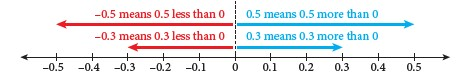## Positive and Negative Decimals

1.4  Positive and Negative Decimals

• Positive decimals are decimals more than $$0$$.
• Negative decimals are decimals less than $$0$$.• The values of two or more decimals can be compared and arranged in ascending order or descending order.

Combined basic arithmetic operations of positive and negative decimals:

 Example Calculate: (i) \begin{aligned} &\space(9.37-0.63)\times(-0.4) \\\\&=8.74\times(-0.4) \\\\&=-3.496. \end{aligned} (ii) \begin{aligned} &\space2.34+3.1\div0.2 \\\\&=2.34+(3.1\div0.2) \\\\&=2.34+15.5 \\\\&=17.84. \end{aligned}